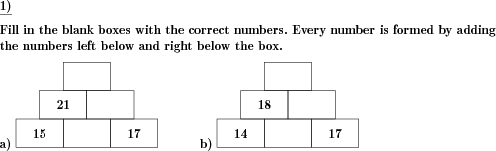Math worksheetsCustom math worksheets at your fingertips

# Details for problem "Number wall sums of whole numbers"

Quickname: 8704

## Summary

In the number wall missing sums have to be added.

## Example## Description

This is a classic number wall problem, where positive natural numbers are summed up ascending through the wall.

The number for each brick is formed by adding the numbers in the brick left below and right below the brick. Some bricks are left blank - these have to be filled in with the correct number. Depending on whether both values below an empty box are given, this may be an addition or a subtraction problem.

The number range for the numbers occuring in the number wall can be specified. The wall height is selectable.

Download free worksheets for this math problem here. There is a second sheet with the solutions. Just click on the respective link.Worksheet 1Solution sheetWorksheet 2Solution sheetWorksheet 3Solution sheet

If you can not see the solution sheets for download, they may be filtered out by an ad blocker that you may have installed. If this is the case, please allow ads for this page and reload the page. The solution sheets will then reappear.

• Do the sample worksheets do not really fit?
• Do you need more math worksheets, with a different level of difficulty?
• Would you like to combine different problems on a worksheet and adjust them to your needs?
• As a teacher, you can put together your own worksheets using the automatically generated math problems provided.
With a free initial credit, you can start creating your own math worksheets in a few minutes.

It does not cost anything to try! Register here, to create custom worksheets now!

## Customization options for this problem

ParameterPossible values
Number of problems1, 2, 3, 4, 5, 6, 7, 8, 9, 10
Number range20, 30, 40, 50, 100, 200, 500, 1000, 10000, 100000
Height of wallthree, four